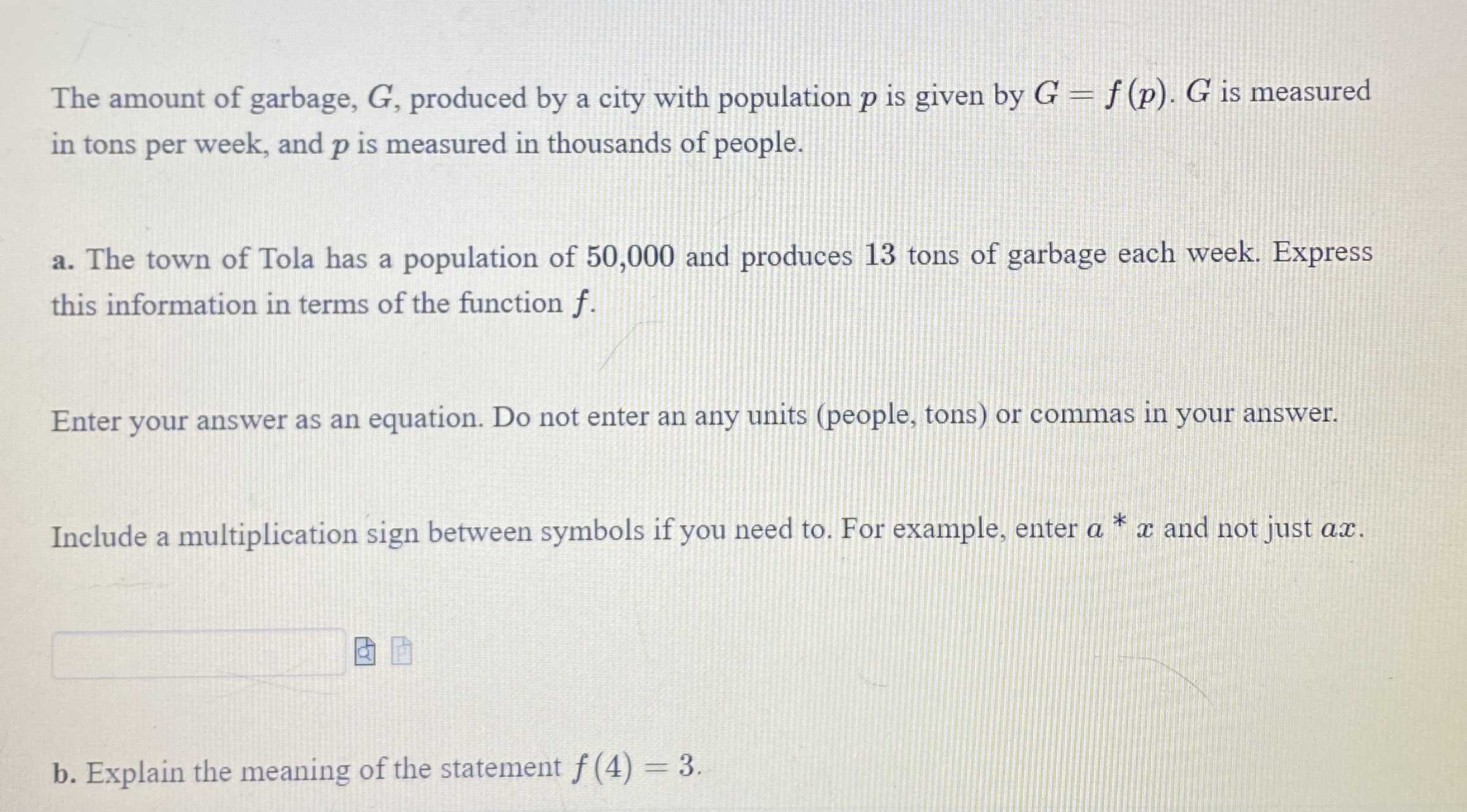### ¿Todavía tienes preguntas de matemáticas?

Pregunte a nuestros tutores expertos
Algebra
PreguntaThe amount of garbage, $$G$$ , produced by a city with population $$p$$ is given by $$G = f ( p ) . G$$ is measured in tons per week, and $$p$$ is measured in thousands of people. a. The town of Tola has a population of $$50,000$$ and produces $$13$$ tons of garbage each week. Express this information in terms of the function $$f$$ . Enter your answer as an equation. Do not enter an any units (people, tons) or commas in your answer. Include a multiplication sign between symbols if you need to. For example, enter $$a ^ { * } x$$ and not just $$a x$$ . b. Explain the meaning of the statement $$f ( 4 ) = 3$$ .

a. f(p)=$$\frac{13}{50} p$$